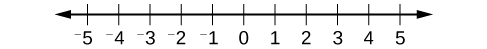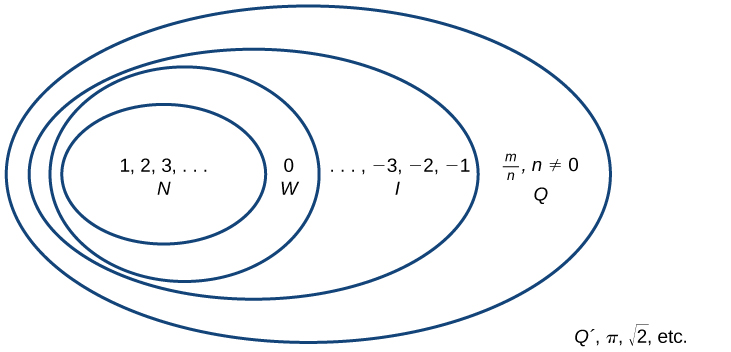## Classifying a Real Number

The numbers we use for counting, or enumerating items, are the natural numbers: 1, 2, 3, 4, 5, and so on. We describe them in set notation as {1, 2, 3, …} where the ellipsis (…) indicates that the numbers continue to infinity. The natural numbers are, of course, also called the counting numbers. Any time we enumerate the members of a team, count the coins in a collection, or tally the trees in a grove, we are using the set of natural numbers. The set of whole numbers is the set of natural numbers plus zero: {0, 1, 2, 3,…}.

The set of integers adds the opposites of the natural numbers to the set of whole numbers: {…-3, -2, -1, 0, 1, 2, 3,…}. It is useful to note that the set of integers is made up of three distinct subsets: negative integers, zero, and positive integers. In this sense, the positive integers are just the natural numbers. Another way to think about it is that the natural numbers are a subset of the integers.

$\begin{array}{lll}{\text{negative integers}}\hfill & {\text{zero}}\hfill & {\text{positive integers}}\\{\dots ,-3,-2,-1,}\hfill & {0,}\hfill & {1,2,3,\dots }\end{array}$

The set of rational numbers is written as $\left\{\frac{m}{n}|m\text{ and }{n}\text{ are integers and }{n}\ne{ 0 }\right\}$. Notice from the definition that rational numbers are fractions (or quotients) containing integers in both the numerator and the denominator, and the denominator is never 0. We can also see that every natural number, whole number, and integer is a rational number with a denominator of 1.

Because they are fractions, any rational number can also be expressed in decimal form. Any rational number can be represented as either:

1. a terminating decimal: $\frac{15}{8}=1.875$, or
2. a repeating decimal: $\frac{4}{11}=0.36363636\dots =0.\overline{36}$

We use a line drawn over the repeating block of numbers instead of writing the group multiple times.

### Example 1: Writing Integers as Rational Numbers

Write each of the following as a rational number.

1. 7
2. 0
3. –8

### Solution

Write a fraction with the integer in the numerator and 1 in the denominator.

1. $7=\frac{7}{1}$
2. $0=\frac{0}{1}$
3. $-8=-\frac{8}{1}$

### Try It 1

Write each of the following as a rational number.

a. 11
b. 3
c. –4

Solution

### Example 2: Identifying Rational Numbers

Write each of the following rational numbers as either a terminating or repeating decimal.

1. $-\frac{5}{7}$
2. $\frac{15}{5}$
3. $\frac{13}{25}$

### Solution

Write each fraction as a decimal by dividing the numerator by the denominator.

1. $-\frac{5}{7}=-0.\overline{714285}$, a repeating decimal
2. $\frac{15}{5}=3$ (or 3.0), a terminating decimal
3. $\frac{13}{25}=0.52$,
a terminating decimal

### Try It 2

Write each of the following rational numbers as either a terminating or repeating decimal.

a. $\frac{68}{17}$
b. $\frac{8}{13}$
c. $-\frac{17}{20}$

Solution

## Irrational Numbers

At some point in the ancient past, someone discovered that not all numbers are rational numbers. A builder, for instance, may have found that the diagonal of a square with unit sides was not 2 or even $\frac{3}{2}$, but was something else. Or a garment maker might have observed that the ratio of the circumference to the diameter of a roll of cloth was a little bit more than 3, but still not a rational number. Such numbers are said to be irrational because they cannot be written as fractions. These numbers make up the set of irrational numbers. Irrational numbers cannot be expressed as a fraction of two integers. It is impossible to describe this set of numbers by a single rule except to say that a number is irrational if it is not rational. So we write this as shown.

{h | h is not a rational number}

### Example 3: Differentiating Rational and Irrational Numbers

Determine whether each of the following numbers is rational or irrational. If it is rational, determine whether it is a terminating or repeating decimal.

1. $\sqrt{25}$
2. $\frac{33}{9}$
3. $\sqrt{11}$
4. $\frac{17}{34}$
5. $0.3033033303333\dots$

### Solution

1. $\sqrt{25}:$ This can be simplified as $\sqrt{25}=5$. Therefore, $\sqrt{25}$ is rational.
2. $\frac{33}{9}:$ Because it is a fraction, $\frac{33}{9}$ is a rational number. Next, simplify and divide.
$\frac{33}{9}=\frac{{{11}\cdot{3}}}{{{3}\cot{3}}}=\frac{11}{3}=3.\overline{6}$

So, $\frac{33}{9}$ is rational and a repeating decimal.

3. $\sqrt{11}:$ This cannot be simplified any further. Therefore, $\sqrt{11}$ is an irrational number.
4. $\frac{17}{34}:$ Because it is a fraction, $\frac{17}{34}$ is a rational number. Simplify and divide.
$\frac{17}{34}=\frac{{1}{\overline{)17}}}{\underset{2}{\overline{)34}}}=\frac{1}{2}=0.5$

So, $\frac{17}{34}$ is rational and a terminating decimal.

5. 0.3033033303333… is not a terminating decimal. Also note that there is no repeating pattern because the group of 3s increases each time. Therefore it is neither a terminating nor a repeating decimal and, hence, not a rational number. It is an irrational number.

### Try It 3

Determine whether each of the following numbers is rational or irrational. If it is rational, determine whether it is a terminating or repeating decimal.

a. $\frac{7}{77}$
b. $\sqrt{81}$
c. $4.27027002700027\dots$
d. $\frac{91}{13}$
e. $\sqrt{39}$

Solution

## Real Numbers

Given any number n, we know that n is either rational or irrational. It cannot be both. The sets of rational and irrational numbers together make up the set of real numbers. As we saw with integers, the real numbers can be divided into three subsets: negative real numbers, zero, and positive real numbers. Each subset includes fractions, decimals, and irrational numbers according to their algebraic sign (+ or –). Zero is considered neither positive nor negative.

The real numbers can be visualized on a horizontal number line with an arbitrary point chosen as 0, with negative numbers to the left of 0 and positive numbers to the right of 0. A fixed unit distance is then used to mark off each integer (or other basic value) on either side of 0. Any real number corresponds to a unique position on the number line.The converse is also true: Each location on the number line corresponds to exactly one real number. This is known as a one-to-one correspondence. We refer to this as the real number line as shown in Figure 1.Figure 1. The real number line

### Example 4: Classifying Real Numbers

Classify each number as either positive or negative and as either rational or irrational. Does the number lie to the left or the right of 0 on the number line?

1. $-\frac{10}{3}$
2. $\sqrt{5}$
3. $-\sqrt{289}$
4. $-6\pi$
5. $0.615384615384\dots$

### Solution

1. $-\frac{10}{3}$ is negative and rational. It lies to the left of 0 on the number line.
2. $\sqrt{5}$ is positive and irrational. It lies to the right of 0.
3. $-\sqrt{289}=-\sqrt{{17}^{2}}=-17$ is negative and rational. It lies to the left of 0.
4. $-6\pi$ is negative and irrational. It lies to the left of 0.
5. $0.615384615384\dots$ is a repeating decimal so it is rational and positive. It lies to the right of 0.

### Try It 4

Classify each number as either positive or negative and as either rational or irrational. Does the number lie to the left or the right of 0 on the number line?

a. $\sqrt{73}$
b. $-11.411411411\dots$
c. $\frac{47}{19}$
d. $-\frac{\sqrt{5}}{2}$
e. $6.210735$

Solution

## Sets of Numbers as Subsets

Beginning with the natural numbers, we have expanded each set to form a larger set, meaning that there is a subset relationship between the sets of numbers we have encountered so far. These relationships become more obvious when seen as a diagram.Figure 2. Sets of numbers.   N: the set of natural numbers   W: the set of whole numbers   I: the set of integers   Q: the set of rational numbers   : the set of irrational numbers

### A General Note: Sets of Numbers

The set of natural numbers includes the numbers used for counting: $\{1,2,3,\dots\}$.

The set of whole numbers is the set of natural numbers plus zero: $\{0,1,2,3,\dots\}$.

The set of integers adds the negative natural numbers to the set of whole numbers: $\{\dots,-3,-2,-1,0,1,2,3,\dots\}$.

The set of rational numbers includes fractions written as $\{\frac{m}{n}|m\text{ and }n\text{ are integers and }n\ne 0\}$.

The set of irrational numbers is the set of numbers that are not rational, are nonrepeating, and are nonterminating: $\{h|h\text{ is not a rational number}\}$.

### Example 5: Differentiating the Sets of Numbers

Classify each number as being a natural number (N), whole number (W), integer (I), rational number (Q), and/or irrational number (Q’).

1. $\sqrt{36}$
2. $\frac{8}{3}$
3. $\sqrt{73}$
4. $-6$
5. $3.2121121112\dots$

### Solution

N W I Q Q’
1. $\sqrt{36}=6$ X X X X
2. $\frac{8}{3}=2.\overline{6}$ X
3. $\sqrt{73}$ X
4. –6 X X
5. $3.2121121112\dots$ X

### Try It 5

Classify each number as being a natural number (N), whole number (W), integer (I), rational number (Q), and/or irrational number (Q’).

a. $-\frac{35}{7}$
b. $0$
c. $\sqrt{169}$
d. $\sqrt{24}$
e. $4.763763763\dots$

Solution